5th Fubon Senior Open Taiwan (Taipei) 2016Wed 15 to Sun 19 June NT 5.6 million @ US\$ 165,000 prize purseChang Gung GCTaipei - Taiwan June 15, 2016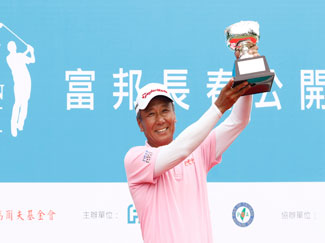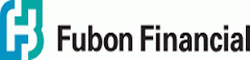Congratulations Champion - Lu Wen-Teh (Taiwan) who returned a 3 day 54 hole score of 207 coming in at 9 under par at the 5th Fubon Senior Open / AST @ Taiwan (Taipei) 2016 15 to 19 June @ Chang Gung GC - US\$ 165,000 prize purse 5th Fubon Senior Open - AST @ Taiwan (Taipei) 2016 3 day Seniors Tournament results 1. - Lu Wen-The (Taiwan) = 68 + 69 + 70 = 207 2. - Masahiro Kuramoto (Japan) = 67 + 71 + 72 = 210 T 3. - Tsuyoshi Yoneyama (Japan) = 71 + 70 + 71 = 212 T 3. - Naoyuki Tamura (Japan) = 69 + 73 + 70 = 212 T 5. - Murugiah (Singapore) = 75 + 68 + 70 = 213 T 5. - Weng Young-Tien (Taiwan) = 72 + 73 + 68 = 213 7. - Lu Chien-Soon (Taiwan) = 69 + 72 + 73 = 214 8. - Richard Backwell (Australia) = 72 + 72 + 72 = 216 T 9. - Hsieh Chin-Sheng (Taiwan) = 217 T 9. - Chen Yun-Mao (Taiwan) = 217 T 9. - Shinichi Akiba (Japan) = 217 T 9. - Jamnian Chitprasong (Thailand) = 217 5th Fubon Senior Open - AST @ Taiwan (Taipei) 2016 2 day Super Seniors Tournament results 1. - Hsieh Min-Nan (Taiwan) = 72 + 74 = 146 T 2. - Wang Chou-Chin (Taiwan) = 70 + 79 = 149 T 2. - Chang Kuo-Jui (Taiwan) = 76 + 73 = 149 Scroll down to view full leader board / results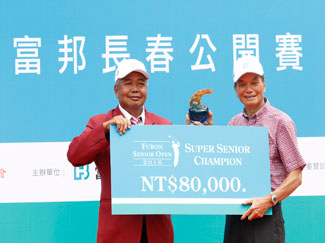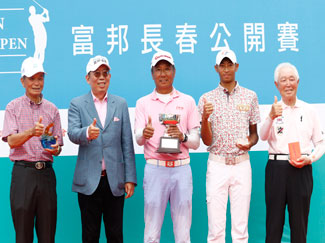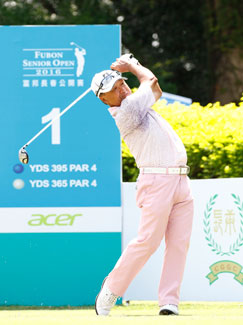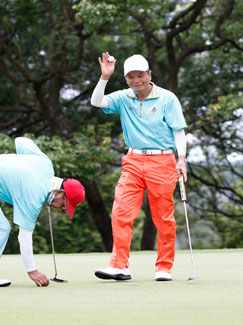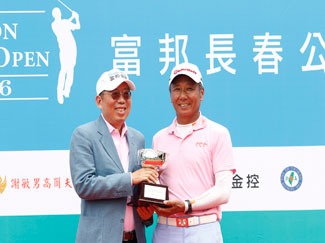5th Fubon Senior Open - AST @ Taiwan (Taipei) 2016 3 day Seniors Tournament results 1. - Lu Wen-The (Taiwan) = 68 + 69 + 70 = 207 2. - Masahiro Kuramoto (Japan) = 67 + 71 + 72 = 210 T 3. - Tsuyoshi Yoneyama (Japan) = 71 + 70 + 71 = 212 T 3. - Naoyuki Tamura (Japan) = 69 + 73 + 70 = 212 T 5. - Murugiah (Singapore) = 75 + 68 + 70 = 213 T 5. - Weng Young-Tien (Taiwan) = 72 + 73 + 68 = 213 7. - Lu Chien-Soon (Taiwan) = 69 + 72 + 73 = 214 8. - Richard Backwell (Australia) = 72 + 72 + 72 = 216 T 9. - Hsieh Chin-Sheng (Taiwan) = 217 T 9. - Chen Yun-Mao (Taiwan) = 217 T 9. - Shinichi Akiba (Japan) = 217 T 9. - Jamnian Chitprasong (Thailand) = 217 T 13. - Chan Chin-Tang (Taiwan) = 218 T 13. - Hsu Shao Min (Taiwan) = 218 T 15. - Chen Jung-Hsin (Taiwan) = 219 T 15. - Chan Wang-Ken (Taiwan) = 219 T 17. - Tim Thelen (USA) = 220 T 17. - Boonchu Ruangkit (Thailand) = 220 T 17. - Wang Ter-Chang (Taiwan) = 220 T 20. - Chou Wen-Chin (Taiwan) = 221 T 20. - Liao Song-Cheng (Taiwan) = 221 22. - Takeshi Sakiyama (Japan) = 222 T 23. - Chang Chin-Chu (Taiwan) = 223 T 23. - Tu Chin-Li (Taiwan) = 223 T 25. - Manas Saengsui (Taiwan) = 224 T 25. - Chen Tsang-Te (Taiwan) = 224 T 25. - Chou Hung-Nan (Taiwan) = 224 T 25. - Lin Te-Ming (Taiwan) = 224 T 29. - Shen Chung-Shyan (Taiwan) = 225 T 29. - Chang Hung-Ta (Taiwan) = 225 T 29. - Dominique Boulet (Hong Kong) = 225 T 29. - Lin Chie-Hsiang (Taiwan) = 225 T 29. - Nobumitsu Yuhara (Japan) = 225 T 34. - Hsu Ten-Lai (Taiwan) = 226 T 34. - Gerry Norquist (USA) = 226 T 34. - Katsumi Kubo (Japan) = 226 T 37. - Chang Mu-Chuan (Taiwan) = 228 T 37. - Chen Chin-Tang (Taiwan) = 228 39. - Chen Chin-Chung (Taiwan) = 229 T 40. - Scott Puzey (USA) = 230 T 40. - Chen Tze-Ming (Taiwan) = 230 T 40. - Lu Chung-Piao (Taiwan) = 230 T 40. - Ahmad Bateman (USA) = 230 T 40. - Huang Shih-Her (Taiwan) = 230 T 40. - Robin Briars (England) = 230 T 40 Yeh Chang-Ming (Taiwan) = 230 T 40. - Roszali Baharuddin (Malaysia) = 230 T 48. - Tsai Chung-Ho (Taiwan) = 231 T 48. - Hiroshi Makino (Japan) = 231 T 50. - Chen Jung-Ching (Taiwan) = 232 T 50. - Lin Chih-Cheng (Taiwan) = 232 T 50. - Peng Cheng-Chun (Taiwan) = 232 T 50. - Mus Deboub (Holland) = 232 T 54. - Chang Kua-Ching (Taiwan) = 233 T 54. - Suthep Boonpimook (Thailand) = 233 T 54. - Cheng Sheng-Yuan (Taiwan) = 233 57. - Kuo I-Liang (Taiwan) = 235 58. - Liao Te-Sen (Taiwan) = 237 59. - Chou Chin-yi (Taiwan) = 241 60. - Chen Kuo-Chun (Taiwan) = 243 61. - Chen Hsi-Shui (Taiwan) = 244 5th Fubon Senior Open - AST @ Taiwan (Taipei) 2016 2 day Super Seniors Tournament results 1. - Hsieh Min-Nan (Taiwan) = 72 + 74 = 146 T 2. - Wang Chou-Chin (Taiwan) = 70 + 79 = 149 T 2. - Chang Kuo-Jui (Taiwan) = 76 + 73 = 149 4. - Hsu Sheng-Chuan (Taiwan) = 74 + 76 = 150 5. - David Hutchison (Taiwan) = 75 + 78 = 153 6. - Mitsuo Hirukawa (Japan) = 155 T 7. - Liu Deng-Chu (Taiwan) = 159 T 7. - Ho Ming-Chung (Taiwan) = 159 T 9. - Yutaka Kondo (Japan) = 160 T 9. - Lu Shui-Chi (Taiwan) = 160 T 9. - Hung Wen-Neng (Taiwan) = 160 T 12. - Kuo Chie-Hsiung (Taiwan) = 161 T 12. - Lee Ming-Hsing (Taiwan) = 161 14. - Lin Ming-Chuan (Taiwan) = 163 15. - Siu Kam-Piu (Hong Kong) = 164 T 16. - Lee Chang-Ken (Taiwan) = 165 T 16. - Uong Chun-Ching (Taiwan) = 165 18. - Chang Chiu-Tung (Taiwan) = 168 19. - Lee Chin-Hsiung (Taiwan) = 169 T 20. - Lu Liang-Wong (Taiwan) = 170 T 20. - Yu Cheng-Chih (Taiwan) = 170 T 22. - Hsieh Chung-Sheng (Taiwan) = 174 T 22. - Lu Chai-Chi (Taiwan) = 174

Results (click on images for enlargement)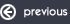Group Photos from5th Fubon Senior Open Taiwan (Taipei) 2016Wed 15 to Sun 19 June NT 5.6 million @ US\$ 165,000 prize pursea full photo review of the day is available here.Copyright © Asian Seniors Tour Pte Ltd 2005 - . All Rights Reserved.